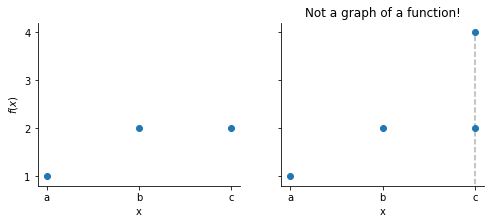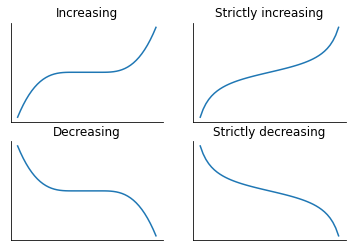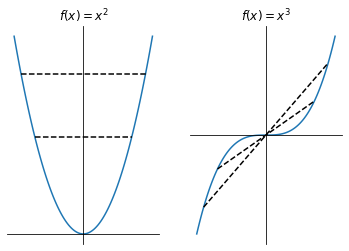# Functions#

Mathematics for Geoscientists

## Basic definitions#

Let us give a somewhat informal definition of a function.

Let $$\mathcal{D}$$ and $$\mathcal{C}$$ be non-empty sets and let $$f$$ be a rule by which every element $$x \in \mathcal{D}$$ is assigned a unique element $$y \in \mathcal{C}$$. The ordered triple $$(\mathcal{D}, \mathcal{C}, f)$$ is called a function (also map or mapping). We write $$f: \mathcal{D} \to \mathcal{C}$$.

Note

What that means is that the following three functions are all different despite their assignment $$f$$ being the same:

\begin{split} \begin{aligned} f_1 : \mathbb{R} \to \mathbb{R}, \quad & f_1(x) = x^2 \\ f_2 : \mathbb{R} \to [0, \infty), \quad & f_2(x) = x^2 \\ f_3 : (- \infty, 0) \to (0, \infty), \quad & f_3(x) = x^2 \end{aligned}\end{split}

Functions $$(\mathcal{D}_1, \mathcal{C}_1, f_1)$$ and $$(\mathcal{D}_2, \mathcal{C}_2, f_2)$$ are equal iff $$\mathcal{D}_1 = \mathcal{D}_2, \mathcal{C}_1 = \mathcal{C}_2, f_1 = f_2$$.

• $$\mathcal{D(f)}$$ is called the domain of the function $$f$$

• $$\mathcal{C(f)}$$ is called the codomain of the function $$f$$

• $$f(x)$$ is the value (sometimes called output) of $$f$$ at point $$x$$

Example:

The figure on the left shows a function where $$a \mapsto f(a) = 1, b \mapsto f(b) = 2, c \mapsto f(c)=2$$. The figure on the right does not represent a function since $$c$$ is assigned both $$2$$ and $$4$$.

## Graph#

The graph of the function is the set

$G(f) := \{ (x, f(x)) : x \in \mathcal{D} \}.$

Therefore, $$G(f) \subseteq \mathcal{D} \times \mathcal{C} = { (d, c) : d \in \mathcal{D}, c \in \mathcal{C} }$$ .

From above examples we have the following graphs:

import matplotlib.pyplot as plt

fig, ax = plt.subplots(1, 2, figsize=(8, 3), sharey=True)

ax.scatter(['a', 'b', 'c'], y = [1, 2, 2])
ax.set_ylabel('$f(x)$')

ax.scatter(['a', 'b', 'c', 'c'], y = [1, 2, 2, 4], zorder=10)
ax.plot(['c', 'c'], [0, 4], '--k', alpha=0.3)
ax.set_title('Not a graph of a function!')

for i in [0, 1]:
ax[i].set_yticks([1, 2, 3, 4])
ax[i].spines['right'].set_visible(False)
ax[i].spines['top'].set_visible(False)
ax[i].set_ylim(0.8, 4.2)
ax[i].set_xlabel('x')

plt.show()The graph on the right is not a graph of a function because two points lie on the vertical line drawn through $$c$$, i.e. $$c$$ is assigned two different values $$f(c)$$. This is a contradiction to our definition of a function. Sometimes this is called the vertical line test.

## Restriction#

Let $$f : \mathcal{D} \to \mathcal{C}$$ be a function and let $$A \subseteq \mathcal{D}$$. We can define a restriction of $$f$$ to the domain of $$A$$ as $$\left.f\right|_A : A \to \mathcal{C}$$ with $$\left.f\right|_A (x) = f(x), \forall x \in A$$.

## Image#

Let $$f : \mathcal{D} \to \mathcal{C}$$ be a function and let $$A \subseteq \mathcal{D}$$. The image of a subset $$A$$ under $$f$$ is the set $$f(A) \subseteq \mathcal{C}$$ such that:

$f(A) := \{ f(x) : x \in A \} \subseteq \mathcal{C}.$

If $$A = \mathcal{D}$$, i.e. the entire domain, we call the set $$f(D)$$ the image or range of $$f$$. In other words, the image is a set of all values that $$f(x)$$ can take for all $$x$$ in the domain.

From our example of a function above (left figure), let us take $$A = \{ a, c \}$$. The image of a subset $$A$$ is then:

$f(A) = \{ f(x) : x \in A \} = \{ \underbrace{f(a)}_{=1}, \underbrace{f(b)}_{=2} \} = \{ 1, 2 \}.$

Similarly for the entire domain:

$f(D) = \{ f(x) : x \in \mathcal{D} \} = \{ \underbrace{f(a)}_{=1}, \underbrace{f(b)}_{=2}, \underbrace{f(c)}_{=2} \} = \{ 1, 2 \}.$

## Inverse image#

Let $$f : \mathcal{D} \to \mathcal{C}$$ be a function and let $$E \subseteq \mathcal{C}$$. The inverse image (or preimage) of $$E$$ under function $$f$$ is the set

$f^{-1}(E) := \{ x \in \mathcal{D} : f(x) \in E \} \subseteq \mathcal{D}.$

If we take $$E = \mathcal{C}$$, then $$f^{-1}(\mathcal{C}) = \mathcal{D}$$. In other words, we are looking for the values $$x \in \mathcal{D}$$ for which $$f(x)$$ takes specified values.

Again from the example above, let us consider $$E_1 = { 1 }, E_2 = { 2 }, E_3 = { 1, 2 }$$:

$\begin{split} f^{-1}(E_1) = \{ x \in \mathcal{D} : f(x) \in E_1 \} = \{ x \in \mathcal{D} : f(x) = 1 \} = \{ a \} \\ f^{-1}(E_2) = \{ x \in \mathcal{D} : f(x) \in E_2 \} = \{ x \in \mathcal{D} : f(x) = 2 \} = \{ b, c \} \end{split}$

Or some may appreciate a more applied example: let $$f: \mathbb{R} \to \mathbb{R}$$ be a function with $$f(x) = x^2$$, then

$\begin{split} f([0, \infty)) = [0, \infty), \qquad f( (- \infty, 0] ) = [0, \infty) \\ f^{-1}([0, \infty)) = \mathbb{R}, \qquad f^{-1}((- \infty, 0)) = \emptyset. \end{split}$

The last point means that there are no $$x \in \mathbb{R}$$ such that $$f(x) = x^2$$ is a negative number.

Or a more applied example: consider $$f: \mathbb{R} \to \mathbb{R}$$ where $$f(x) = x - 5$$ (we can simply write $$x \mapsto x - 5$$) and $$A = (-3, 1]$$. Let us find the preimage $$f^{-1}(A)$$ graphically and by calculating it.

\begin{split} \begin{aligned} f^{-1}(A) & = \{ x \in \mathbb{R} : f(x) \in A \} \\ & = \{ x \in \mathbb{R} : x - 5 \in (-3, 1] \} \\ & = \{ x \in \mathbb{R} : -3 < x - 5 \leq -1 \} \end{aligned} \end{split}
\begin{split} \begin{aligned} x - 5 > -3 \quad & \text{and} \quad x - 5 \leq -1 \\ x > 2 \quad & \text{and} \quad x \leq 4 \end{aligned}\end{split}
\begin{split} \begin{aligned} & \implies x \in (2, 4] \\ & \implies f^{-1}(A) = (2, 4] \end{aligned}\end{split}

This agrees with our findings on the graph.

## Real functions#

If $$\mathcal{D}$$ and $$\mathcal{C}$$ are subsets of $$\mathbb{R}$$ then we call a function $$f : \mathcal{D} \to \mathcal{C}$$ a real-valued function of a real variable or, simply, a real function.

The largest subset of $$\mathbb{R}$$ for which the function $$f$$ is defined is called the natural domain.

Examples. We give further examples in the notebook elementary_functions.

Function

Natural domain

Image

$$f(x) = x^2$$

$$\mathbb{R}$$

$$[0, + \infty)$$

$$f(x)=\sqrt{x}$$

$$[0, + \infty)$$

$$[0, + \infty)$$

$$f(x) = \frac{1}{x}$$

$$\mathbb{R} \backslash { 0 }$$

$$(- \infty, 0) \cup (0, + \infty)$$

### Monotonic functions#

Many useful properties of real functions can easily be seen on the graph of the function. One of those is whether a function is monotonic, i.e. whether it is always increasing or decreasing on the whole domain. For a function $$f : A \to \mathbb{R}, A \subseteq \mathbb{R}$$, we say that it is:

• increasing on $$A$$ if $$(x_1 < x_2) \Rightarrow (f(x_1) \leq f(x_2))$$ for all $$x_1, x_2 \in A$$

• strictly increasing on $$A$$ if $$(x_1 < x_2) \Rightarrow (f(x_1) < f(x_2))$$ for all $$x_1, x_2 \in A$$

• decreasing on $$A$$ if $$(x_1 < x_2) \Rightarrow (f(x_1) \geq f(x_2))$$ for all $$x_1, x_2 \in A$$

• strictly decreasing on $$A$$ if $$(x_1 < x_2) \Rightarrow (f(x_1) > f(x_2))$$ for all $$x_1, x_2 \in A$$

import numpy as np

def f(x):
if x <= 0:
y = x**3 + 1
elif x <= 1:
y = 1
else:
y = (x-1)**3 + 1

return y

titles = [['Increasing', 'Strictly increasing'],
['Decreasing', 'Strictly decreasing']]

x1 = np.linspace(-2, 3, 50)
x2 = np.linspace(-1.3, 1.3, 50)

fig, ax = plt.subplots(2, 2)

ax[0, 0].plot(x1, list(map(f, x1)))
ax[0, 1].plot(x2, np.tan(x2))
ax[1, 0].plot(x1, -np.array(list(map(f, x1))))
ax[1, 1].plot(x2, -np.tan(x2))

for i in range(2):
for j in range(2):
ax[i, j].spines['right'].set_visible(False)
ax[i, j].spines['top'].set_visible(False)
ax[i, j].set_title(titles[i][j])
ax[i, j].set_xticks([])
ax[i, j].set_yticks([])

plt.show()### Even and odd functions#

Even and odd functions satisfy particular symmetry relations. A function $$f : A \to \mathbb{R}, A \subseteq \mathbb{R}$$ is:

• even on $$A$$ if $$f(-x) = f(x)$$ for all $$x \in A$$

• odd on $$A$$ if $$f(-x) = -f(x)$$ for all $$x \in A$$

The names ‘even’ and ‘odd’ originate from the power function $$f(x) = x^n$$, which is even for even $$n$$ and odd for odd $$n$$. For example, $$f(x)=x^2$$ and $$f(x)=x^3$$ below:

x = np.linspace(-2, 2, 100)
y1 = x**2
y2 = x**3 / 2

fig, ax = plt.subplots(1, 2)

ax.plot(x, y1)
ax.plot(x, y2)

ax.plot([-1.4, 1.4], [1.4**2, 1.4**2], '--k')
ax.plot([-1.8, 1.8], [1.8**2, 1.8**2], '--k')
ax.plot([-1.4, 1.4], [-1.4**3 / 2, 1.4**3 /2], '--k')
ax.plot([-1.8, 1.8], [-1.8**3 / 2, 1.8**3 /2], '--k')

ax.set_title(r'$f(x) = x^2$')
ax.set_title(r'$f(x) = x^3$')

for i in range(2):
ax[i].spines['left'].set_position('zero')
ax[i].spines['bottom'].set_position('zero')
ax[i].spines['right'].set_visible(False)
ax[i].spines['top'].set_visible(False)
ax[i].set_yticks([])
ax[i].set_xticks([])

plt.show()